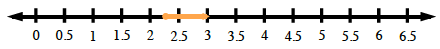### Home > PC > Chapter 7 > Lesson 7.2.2 > Problem7-56

7-56.

Express the solution set using inequality notation and sketch the set on a number line.

1. $4x + 3 ≥ 12$1. $−6 < \frac { 1 } { 2 }x + 1 < 8$

Change the inequality sign if you divide or multiply both sides by a negative or switch the variable to the opposite side.